Python for Computing

Python for Computing

There are many online resources for learning Python. A good place to start is the documentation provided by the Python Software Foundation, located here: https://www.python.org/doc/

This chapter focuses on how to use Python within a Jupyter notebook. Doing simple calculations in Python is very straightforward. However, once you try to do something complex, there are a few tricks to learn. In particular, how to get plots to appear in the notebook, how to do animations, and a few other niceties.

Python 2 or 3

Please use Python 3. That is the modern version of the language.

Many people recommend using Python 2 because some software libraries (particularly in scientific computing) have not been converted yet to the new version of Python. This can be a problem, but really, Python 3 has been around for almost a decade (released in Dec 2008) so get with the game, folks!

For the record, Apple’s Mac OS X still is only equipped with Python 2. You can upgrade from the usual sources (e.g. Anaconda). This only makes a difference if you want to run your code locally.

How different are the two versions? Not much. The command 23 returns 0 in Python 2 (integer division) while 23 returns .66666666666 in Python 3 (floating point division). And printing is a bit different. And there is enough new, good stuff in Python 3 that you really should use it

Note

Plotting in a Python notebook

Three things before you can plot.

• you tell Jupyter that you want plots to appear inline
• you load in numerical Python so you can deal with numerical arrays

This is done with the following code:

%matplotlib inline
import numpy as np
import matplotlib.pyplot as plt


You don’t need to use the “as np” or the “as plt” but it helps to keep the namespace clean. So when you call functions like “sin” you need to say where it comes from, as in “np.sin”

Now, to plot a few numbers, you just call the plot function, with the numbers as an array:

plt.plot([1,4,9,16,25])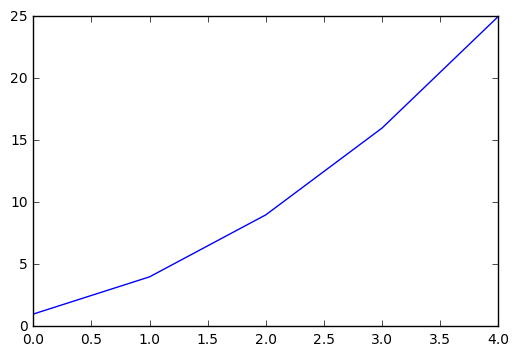You can plot a piece of the sine function in a similar manner, passing a list of numbers to the sin function:

plt.plot(np.sin([0,.1,.2,.3,.4,.5,.6,.7,.8,.9,1.0]))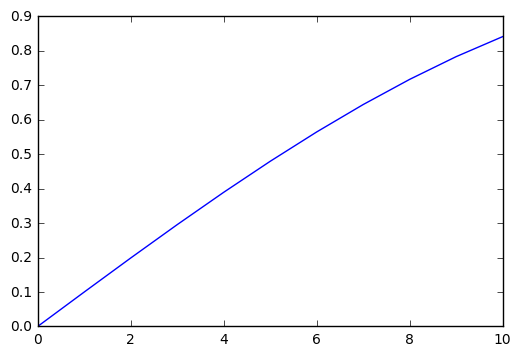Usually you want to plot x and y values together, which you can do as follows:

x = [0,.1,.2,.3,.4,.5,.6,.7,.8,.9,1.0,1.1,1.2,1.3,1.4,1.5,1.6];
y = np.sin(x);
plt.plot(x,y)Animating Plots

We can use html5 for animation, as suggested in this web page.

This seems to be the modern way to do animation in Python.

First step is to initialize some things in Python.

• we need %matplotlib inline to get things to plot right on the notebook
• we need numpy for the math
• we need matplotlib for plotting
• we need animation from matplotlib, and HTML from iPython.display to show the animations

We get all these with the following imports:

%matplotlib inline
import numpy as np
import matplotlib.pyplot as plt
from matplotlib import animation, rc
from IPython.display import HTML


We now have four steps to get an animated plot

• set up the figure frame
• define the initializing function
• define the function that draws each frame of the animation
• call the animator function, which creates all the frames and saves them for you

Then we are ready to call “HTML” to display the animation.

# First set up the figure, the axis, and the plot element we want to animate
fig, ax = plt.subplots()

ax.set_xlim(( 0, 2))
ax.set_ylim((-2, 2))

line, = ax.plot([], [], lw=2)
# NOTE: THIS WILL DISPLAY JUST AN EMPTY BOX.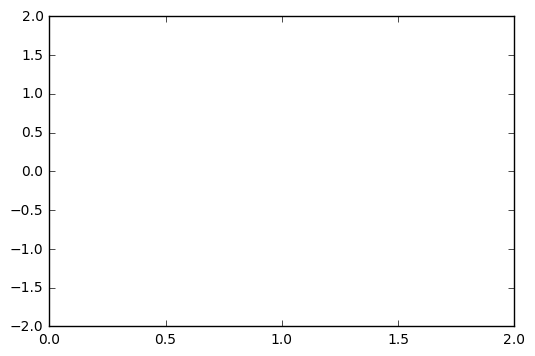Initialization function: plot the background of each frame

def init():
line.set_data([], [])
return (line,)


Animation function. This is called sequentially

def animate(i):
x = np.linspace(0, 2, 1000)
y = np.sin(2 * np.pi * (x - 0.01 * i))
line.set_data(x, y)
return (line,)


Call the animator. blit=True means only re-draw the parts that have changed.

anim = animation.FuncAnimation(fig, animate, init_func=init,
frames=100, interval=20, blit=True)

HTML(anim.to_html5_video())


Anytime you need the animation, you can call it up at will, with the following code:

# equivalent to rcParams['animation.html'] = 'html5'
rc('animation', html='html5')


Data Analysis

A simple demo with pandas in Python

This notebook is based on course notes from Lamoureux’s course Math 651 at the University of Calgary, Winter 2016.

This was an exercise to try out some resource in Python. Specifically, we want to scrape some data from the web concerning stock prices, and display in a Panda. Then do some basic data analysis on the information.

We take advantage of the fact that there is a lot of financial data freely accessible on the web, and lots of people post information about how to use it.

How to access real data from the web and apply data analysis tools.

I am using the book Python for Data Analysis by Wes McKinney as a reference for this section.

The point of using Python for this is that a lot of people have created good code to do this.

The pandas name comes from Panel Data, an econometrics terms for multidimensional structured data sets, as well as from Python Data Analysis.

The dataframe objects that appear in pandas originated in R. But apparently they have more functionality in Python than in R.

I will be using PYLAB as well in this section, so we can make use of NUMPY and MATPLOTLIB.

Accessing financial data

For free, historical data on commodities like Oil, you can try this site: http://www.databank.rbs.com This site will download data directly into spreadsheets for you, plot graphs of historical data, etc. Here is an example of oil prices (West Texas Intermediate), over the last 15 years. Look how low it goes…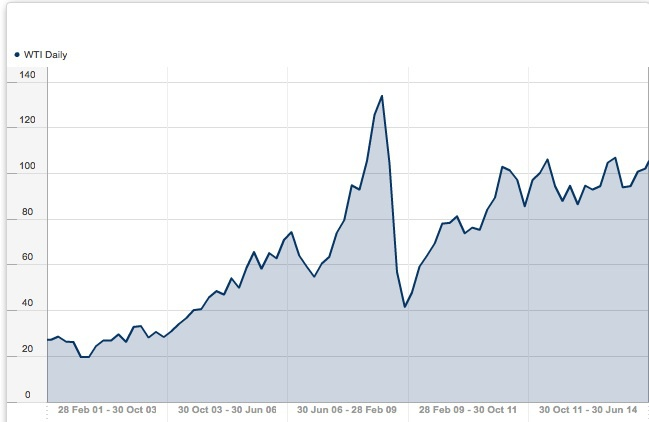Yahoo supplies current stock and commodity prices. Here is an interesting site that tells you how to download loads of data into a csv file. http://www.financialwisdomforum.org/gummy-stuff/Yahoo-data.htm

Here is another site that discusses accessing various financial data sources. http://quant.stackexchange.com/questions/141/what-data-sources-are-available-online

To get away from the highly contentious issues of oil prices and political parties, let’s look at some simple stock prices – say Apple and Microsoft. We can import some basic webtools to get prices directly from Yahoo.

# Get some basic tools
%pylab inline
from pandas import Series, DataFrame
import pandas as pd
import pandas.io.data as web


# Here are apple and microsoft closing prices since 2001
subplot(2,1,1)
plot(aapl)
subplot(2,1,2)
plot(msft)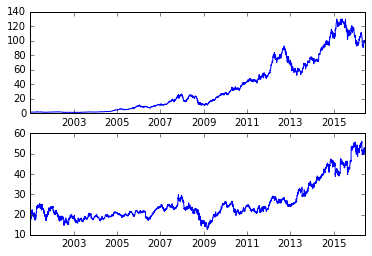aapl

Date
2001-01-02      0.978015
2001-01-03      1.076639
2001-01-04      1.121841
2001-01-05      1.076639
2001-01-08      1.088967
2001-01-09      1.130060
2001-01-10      1.088967
2001-01-11      1.183481
2001-01-12      1.130060
2001-01-16      1.125950
2001-01-17      1.105404
2001-01-18      1.228683
2001-01-19      1.282104
2001-01-22      1.265667
2001-01-23      1.347853
2001-01-24      1.347853
2001-01-25      1.310869
2001-01-26      1.286213
2001-01-29      1.425930
2001-01-30      1.430039
2001-01-31      1.421820
2001-02-01      1.388946
2001-02-02      1.356072
2001-02-05      1.327306
2001-02-06      1.388946
2001-02-07      1.364290
2001-02-08      1.364290
2001-02-09      1.257448
2001-02-12      1.294432
2001-02-13      1.257448
...
2016-05-04     93.620002
2016-05-05     93.239998
2016-05-06     92.720001
2016-05-09     92.790001
2016-05-10     93.419998
2016-05-11     92.510002
2016-05-12     90.339996
2016-05-13     90.519997
2016-05-16     93.879997
2016-05-17     93.489998
2016-05-18     94.559998
2016-05-19     94.199997
2016-05-20     95.220001
2016-05-23     96.430000
2016-05-24     97.900002
2016-05-25     99.620003
2016-05-26    100.410004
2016-05-27    100.349998
2016-05-31     99.860001
2016-06-01     98.459999
2016-06-02     97.720001
2016-06-03     97.919998
2016-06-06     98.629997
2016-06-07     99.029999
2016-06-08     98.940002
2016-06-09     99.650002
2016-06-10     98.830002
2016-06-13     97.339996
2016-06-14     97.459999
2016-06-15     97.139999


Let’s look at the changes in the stock prices, normalized as a percentage

aapl_rets = aapl.pct_change()
msft_rets = msft.pct_change()
subplot(2,1,1)
plot(aapl_rets)
subplot(2,1,2)
plot(msft_rets)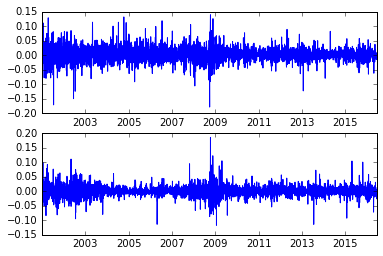Let’s look at the correlation between these two series

pd.rolling_corr(aapl_rets, msft_rets, 250).plot()

/opt/conda/envs/python2/lib/python2.7/site-packages/ipykernel/__main__.py:2: FutureWarning: pd.rolling_corr is deprecated for Series and will be removed in a future version, replace with
Series.rolling(window=250).corr(other=<Series>)
from ipykernel import kernelapp as appGetting fancy.

Now, we can use some more sophisticated statistical tools, like least squares regression. However, I had to do some work to get Python to recognize these items. But I didn’t work too hard, I just followed the error messages.

It became clear that I needed to go back to a terminal window to load in some packages. The two commands I had to type in were

• pip install statsmodels
• pip install patsy

‘pip’ is an ‘python installer package’ that install packages of code onto your computer (or whatever machine is running your python). The two packages ‘statsmodels’ and ‘patsy’ are assorted statistical packages. I don’t know much about them, but they are easy to find on the web.

# We may also try a least square regression, also built in as a panda function
model = pd.ols(y=aapl_rets, x={'MSFT': msft_rets},window=256)

/opt/conda/envs/python2/lib/python2.7/site-packages/ipykernel/__main__.py:2: FutureWarning: The pandas.stats.ols module is deprecated and will be removed in a future version. We refer to external packages like statsmodels, see some examples here: http://statsmodels.sourceforge.net/stable/regression.html
from ipykernel import kernelapp as app
model.beta

MSFT intercept
Date
2002-01-14 0.796463 0.000416
2002-01-15 0.788022 0.000417
2002-01-16 0.789784 0.000191
2002-01-17 0.802081 0.000600
2002-01-18 0.793941 0.000671
2002-01-22 0.796478 0.000718
2002-01-23 0.797909 0.001172
2002-01-24 0.786143 0.000960
2002-01-25 0.781526 0.001102
2002-01-28 0.782449 0.001064
2002-01-29 0.782113 0.001196
2002-01-30 0.764488 0.001069
2002-01-31 0.784935 0.001246
2002-02-01 0.784775 0.001254
2002-02-04 0.774178 0.001252
2002-02-05 0.781460 0.001383
2002-02-06 0.781529 0.001354
2002-02-07 0.792091 0.001506
2002-02-08 0.785451 0.001019
2002-02-11 0.788570 0.001084
2002-02-12 0.793316 0.001001
2002-02-13 0.796715 0.001117
2002-02-14 0.796227 0.001075
2002-02-15 0.801823 0.001176
2002-02-19 0.804453 0.000885
2002-02-20 0.814666 0.001097
2002-02-21 0.830058 0.000799
2002-02-22 0.818041 0.001174
2002-02-25 0.822848 0.001161
2002-02-26 0.821503 0.001248
... ... ...
2016-05-04 0.634886 -0.001187
2016-05-05 0.632351 -0.001121
2016-05-06 0.631153 -0.001282
2016-05-09 0.631186 -0.001277
2016-05-10 0.627805 -0.001240
2016-05-11 0.632612 -0.001326
2016-05-12 0.629215 -0.001440
2016-05-13 0.626302 -0.001429
2016-05-16 0.631538 -0.001302
2016-05-17 0.629171 -0.001258
2016-05-18 0.629934 -0.001218
2016-05-19 0.626321 -0.001243
2016-05-20 0.627607 -0.001232
2016-05-23 0.625496 -0.001210
2016-05-24 0.624308 -0.001229
2016-05-25 0.625938 -0.001186
2016-05-26 0.625857 -0.001192
2016-05-27 0.628037 -0.001277
2016-05-31 0.624431 -0.001256
2016-06-01 0.623145 -0.001322
2016-06-02 0.623414 -0.001335
2016-06-03 0.620833 -0.001279
2016-06-06 0.621354 -0.001256
2016-06-07 0.621346 -0.001237
2016-06-08 0.621420 -0.001246
2016-06-09 0.620108 -0.001200
2016-06-10 0.620255 -0.001216
2016-06-13 0.619448 -0.001207
2016-06-14 0.618853 -0.001180
2016-06-15 0.618974 -0.001180

3631 rows × 2 columns

model.beta['MSFT'].plot()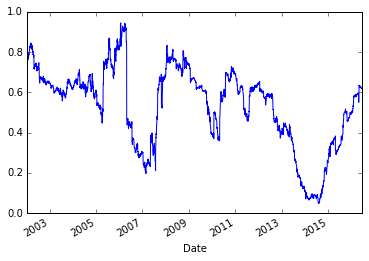Those two graphs looked similar. Let’s plot them together

subplot(2,1,1)
pd.rolling_corr(aapl_rets, msft_rets, 250).plot()
title('Rolling correlations')
subplot(2,1,2)
model.beta['MSFT'].plot()
title('Least squaresn model')

/opt/conda/envs/python2/lib/python2.7/site-packages/ipykernel/__main__.py:3: FutureWarning: pd.rolling_corr is deprecated for Series and will be removed in a future version, replace with
Series.rolling(window=250).corr(other=<Series>)
app.launch_new_instance()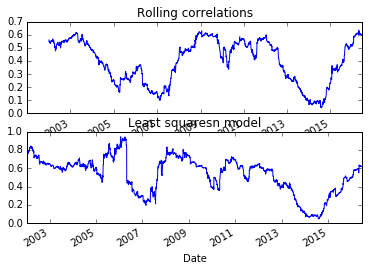more stocks

There is all kinds of neat info on the web. Here is the SPY exchange-traded fund, which tracks the S&P 500 index.

px = web.get_data_yahoo('SPY')['Adj Close']*10
px

Date
2010-01-04     998.08658
2010-01-05    1000.72861
2010-01-06    1001.43318
2010-01-07    1005.66052
2010-01-08    1009.00712
2010-01-11    1010.41626
2010-01-12    1000.99287
2010-01-13    1009.44749
2010-01-14    1012.17761
2010-01-15    1000.81670
2010-01-19    1013.32249
2010-01-20    1003.01842
2010-01-21     983.73128
2010-01-22     961.80210
2010-01-25     966.73395
2010-01-26     962.68278
2010-01-27     967.26241
2010-01-28     956.16569
2010-01-29     945.77354
2010-02-01     960.48105
2010-02-02     972.10617
2010-02-03     967.26241
2010-02-04     937.40701
2010-02-05     939.34454
2010-02-08     932.56318
2010-02-09     944.27638
2010-02-10     942.42694
2010-02-11     952.29063
2010-02-12     951.49804
2010-02-16     966.46975
...
2016-05-04    2050.09995
2016-05-05    2049.70001
2016-05-06    2057.20001
2016-05-09    2058.89999
2016-05-10    2084.49997
2016-05-11    2065.00000
2016-05-12    2065.59998
2016-05-13    2047.59995
2016-05-16    2067.79999
2016-05-17    2048.50006
2016-05-18    2049.10004
2016-05-19    2041.99997
2016-05-20    2054.90005
2016-05-23    2052.10007
2016-05-24    2078.69995
2016-05-25    2092.79999
2016-05-26    2093.39996
2016-05-27    2102.40005
2016-05-31    2098.39996
2016-06-01    2102.70004
2016-06-02    2109.10004
2016-06-03    2102.79999
2016-06-06    2113.50006
2016-06-07    2116.79993
2016-06-08    2123.69995
2016-06-09    2120.80002
2016-06-10    2100.70007
2016-06-13    2084.49997
2016-06-14    2080.39993
2016-06-15    2077.50000

plot(px)Scientific Computing in Python

This is a sample of doing some real scientific computing in Python.

We refer to some ideas in the book “Python for Scientists” by John M. Stewart. Specifically, we want to explore a numerical solver for ordinary differential equations (ODEs), called ODEint. This solver is based on lsoda, a Fortran package from Lawrence Livermore Labs that is a reliable workhorse for solving these difficult problems.

We look at four different ODEs that are interesting, and numerically challenging. They are:

• the harmonic oscillator (or linear pendulum)
• the non-linear pendulum
• the Lorenz equation, which is the model for chaotic behaviour
• the van der Pols equation, which is an example of a stiff ODE with periodic behaviour.

We do some basic tests of an ODE numerical solver, to test its accuracy, and to consider whether our asymptotic analysis of a pendulum is any good.

As in previous examples, we must start with code to tell the Notebook to plot items inline, and load in packages to do numerical computations (numpy), scientific computation (scipy) and plotting (matplotlib).

%matplotlib inline
import numpy as np
import matplotlib.pyplot as plt
from scipy.integrate import odeint # This is the numerical solver


We start with a second order linear equation, that has the usual harmonic oscillator solutions.

$$y”(t) + \omega^2 y(t) = 0, \qquad y(0) = 1, y’(0) = 0.$$

To put this into the numerical solver, we need to reformulate as a 2 dimensional, first order system: $$\mathbf{y} = (y,y’)^T, \qquad \mathbf{y}‘(t) = (y’, -\omega^2 y)^T.$$ Here is a simple code snippet that solves the problem numerically.

def rhs(Y,t,omega):  # this is the function of the right hand side of the ODE
y,ydot = Y
return ydot,-omega*omega*y

t_arr=np.linspace(0,2*np.pi,101)
y_init =[1,0]
omega = 2.0
y_arr=odeint(rhs,y_init,t_arr, args=(omega,))
y,ydot = y_arr[:,0],y_arr[:,1]
plt.ion()
plt.plot(t_arr,y,t_arr,ydot)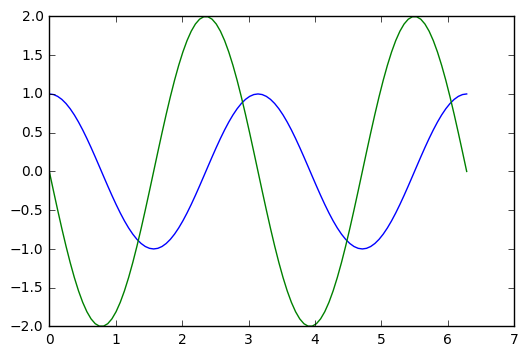Let’s draw a phase portrait, plotting y and ydot together

plt.plot(y,ydot)
plt.title("Solution curve when omega = %4g" % omega)
plt.xlabel("y values")
plt.ylabel("ydot values")Now, I would like to test how accurate this numerical code is, by comparing the exact solution with the numerical solution. The exact solution is given by the initial values of y_init, and omega, and involves cosines and sines.

t_arr=np.linspace(0,2*np.pi,101)
y_init =[1,0]
omega = 2.0
y_exact = y_init*np.cos(omega*t_arr) + y_init*np.sin(omega*t_arr)/omega
ydot_exact = -omega*y_init*np.sin(omega*t_arr) + y_init*np.cos(omega*t_arr)
y_arr=odeint(rhs,y_init,t_arr, args=(omega,))
y,ydot = y_arr[:,0],y_arr[:,1]
plt.plot(t_arr,y,t_arr,y_exact)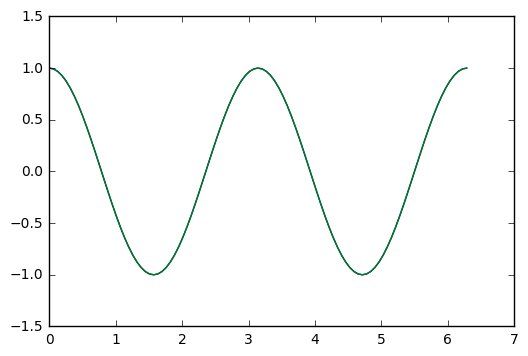We plot the difference

plt.plot(t_arr,y-y_exact)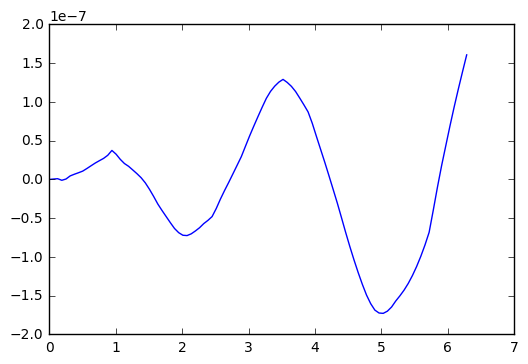So, in the test I did above, we see an error that oscillates and grows with time, getting to about size 2x 10^(-7). Which is single precision accuracy.

Now, apparently ODEint figures out good step sizes on its own. Let’s try running the code again, with different number of steps in the t-variable.

numsteps=1000001  # adjust this parameter
y_init =[1,0]
omega = 2.0
t_arr=np.linspace(0,2*np.pi,numsteps)
y_exact = y_init*np.cos(omega*t_arr) + y_init*np.sin(omega*t_arr)/omega
ydot_exact = -omega*y_init*np.sin(omega*t_arr) + y_init*np.cos(omega*t_arr)
y_arr=odeint(rhs,y_init,t_arr, args=(omega,))
y,ydot = y_arr[:,0],y_arr[:,1]
plt.plot(t_arr,y-y_exact)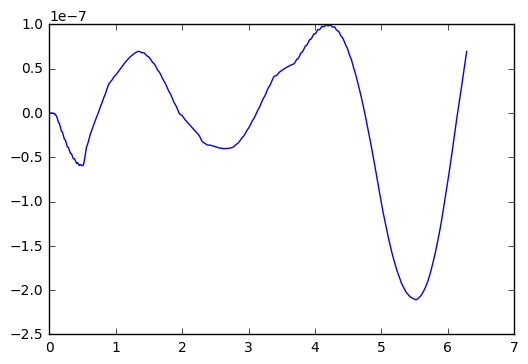Okay, I went up to one million steps, and the error only reduced to about 1.0x10^(-7). Not much of an improvement.

Let’s try another experiment, where we go for a really long time. Say 100 time longer than the example above.

numsteps=100001  # adjust this parameter
y_init =[1,0]
omega = 2.0
t_arr=np.linspace(0,2*1000*np.pi,numsteps)
y_exact = y_init*np.cos(omega*t_arr) + y_init*np.sin(omega*t_arr)/omega
ydot_exact = -omega*y_init*np.sin(omega*t_arr) + y_init*np.cos(omega*t_arr)
y_arr=odeint(rhs,y_init,t_arr, args=(omega,))
y,ydot = y_arr[:,0],y_arr[:,1]
plt.plot(t_arr,y-y_exact)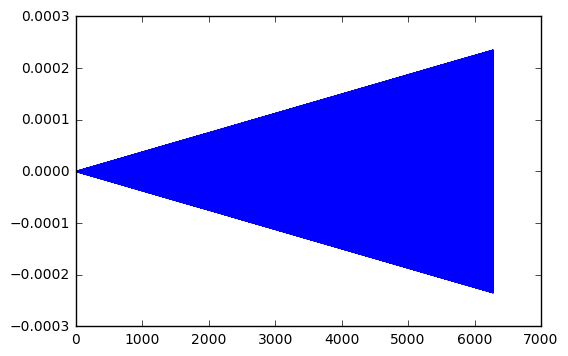Interesting. My little test show the error grow linearly with the length of time. In the first time, 2x10^(-7). For 10 times longer, 2x10^(-6). For 100 times longer, 2x10^(-5). And so one.

A more subtle question: are these amplitude errors, or phase errors, or perhaps a combination?

p1=np.size(t_arr)-1
p0=p1-100
plt.plot(t_arr[p0:p1],y[p0:p1],t_arr[p0:p1],y_exact[p0:p1])plt.plot(t_arr[p0:p1],y[p0:p1]-y_exact[p0:p1])Ah ha! This looks like the negative derivative of the solution, which indicates we have a phase error. Because with phase error, we see the difference
$$\cos(t + \delta) - \cos(t) \approx -\delta\cdot \sin(t)$$
while with an amplitude error, we would see
$$(1+\delta)\cos(t) - \cos(t) \approx \delta\cdot \cos(t).$$

Let’s plot the soluton and the error difference, to see if the derivative zeros line up with peaks.

plt.plot(t_arr[p0:p1],y_exact[p0:p1],t_arr[p0:p1],3000*(y[p0:p1]-y_exact[p0:p1]))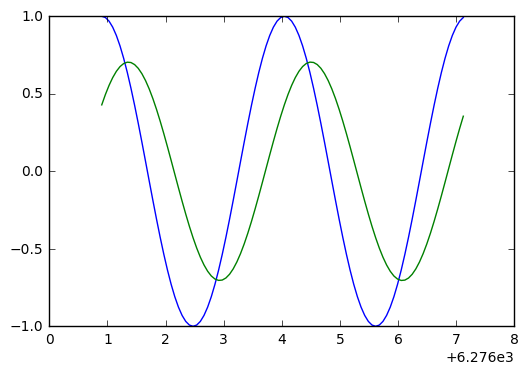Looking at the above, we see they don’t quite line up. So a bit of phase error, a bit of amplitude error.

The non-linear pendulum

Well, it’s not to hard to generalize this stuff to the non-linear pendulum, where the restoring force has a sin(t) in it. We want to observe the period changes with the initial conditions (a bigger swing has a longer period).

The differential equation is:

$$y”(t) + \omega^2 \sin(y(t)) = 0, \qquad y(0) = \epsilon, y’(0) = 0.$$

To put this into the numerical solver, we need to reformulate as a 2 dimensional, first order system:

$$\mathbf{y} = (y,y’)^T, \qquad \mathbf{y}‘(t) = (y’, -\omega^2 \sin(y))^T.$$

Here is a simple code snippet that solves the problem numerically.

def rhsSIN(Y,t,omega):  # this is the function of the right hand side of the ODE
y,ydot = Y
return ydot,-omega*omega*np.sin(y)

omega = .1   # basic frequency
epsilon = .5 # initial displacement, in radians (try 0 to .5)
velocity = 0 # try values from 0 to .2
t_arr=np.linspace(0,2*100*np.pi,1001)
y_init =[epsilon,velocity]

# we first set up the exact solution for the linear oscillator
y_exact = y_init*np.cos(omega*t_arr) + y_init*np.sin(omega*t_arr)/omega
ydot_exact = -omega*y_init*np.sin(omega*t_arr) + y_init*np.cos(omega*t_arr)
y_arr=odeint(rhsSIN,y_init,t_arr, args=(omega,))
y,ydot = y_arr[:,0],y_arr[:,1]
plt.ion()
plt.plot(t_arr,y,t_arr,y_exact)With epsilon = 0.1 (radians, which is about 5.7 degrees), it is hard to see a period shift. With epsilon = 0.5 (radians, which just under 30 degrees), we clearly see a shift after ten cycles of oscillation.

How big is the shift? Can we figure this out easily with numerics? And how does it compare with the estimate given by the Poincare-Lindstedt method?

It is kind of fun to set this up with epsilon = pi, which corresponds to an inverted pendulum. The pendulum sits upside down for a while, then slowly moves away from this unstable equilibrium point. The behaviour is very different than the linear harmonic oscillator. We show the example in the next cell.

omega = .1   # basic frequency
epsilon = np.pi+.0001 # initial displacement, in radians (try 0 to .5)
velocity = 0 # try values from 0 to .2
t_arr=np.linspace(0,2*100*np.pi,1001)
y_init =[epsilon,velocity]

# we first set up the exact solution for the linear oscillator
y_exact = y_init*np.cos(omega*t_arr) + y_init*np.sin(omega*t_arr)/omega
ydot_exact = -omega*y_init*np.sin(omega*t_arr) + y_init*np.cos(omega*t_arr)
y_arr=odeint(rhsSIN,y_init,t_arr, args=(omega,))
y,ydot = y_arr[:,0],y_arr[:,1]
plt.ion()
plt.plot(t_arr,y,t_arr,y_exact)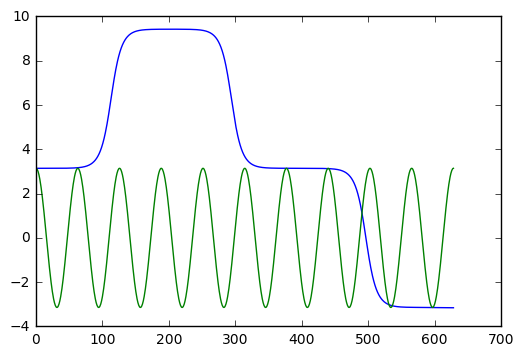The Lorenz equation

You’ve probably heard of the butterfly effect (it’s even a movie). The idea is that weather can demonstrate chaotic behaviour, so that the flap of a butterfly wing in Brazil could eventually result in a tornado in Alabama.

Lorenz was studying a simplified model for weather, given by a system of three simple ODEs:

$$x’ = \sigma(y-x), \quad y’=\rho x - y - xz, \quad z’ = xy - \beta z$$

where $x,y,z$ are functions of time, and $\sigma, \rho,\beta$ are fixed constants.

When $\rho$ is small, the behaviour is quite predictable. But when $\rho$ gets better than about 24.7, you get strange, aperiodic behaviour.

Here is some code that demonstrates the behaviour. We also include 3D plots

def rhsLZ(u,t,beta,rho,sigma):
x,y,z = u
return [sigma*(y-x), rho*x-y-x*z, x*y-beta*z]

sigma = 10.0
beta = 8.0/3.0
rho1 = 29.0
rho2 = 28.8  # two close values for rho give two very different curves

u01=[1.0,1.0,1.0]
u02=[1.0,1.0,1.0]

t=np.linspace(0.0,50.0,10001)
u1=odeint(rhsLZ,u01,t,args=(beta,rho1,sigma))
u2=odeint(rhsLZ,u02,t,args=(beta,rho2,sigma))

x1,y1,z1=u1[:,0],u1[:,1],u1[:,2]
x2,y2,z2=u2[:,0],u2[:,1],u2[:,2]

from mpl_toolkits.mplot3d import Axes3D

plt.ion()
fig=plt.figure()
ax=Axes3D(fig)
ax.plot(x1,y1,z1,'b-')
ax.plot(x2,y2,z2,'r:')
ax.set_xlabel('x')
ax.set_ylabel('y')
ax.set_zlabel('z')
ax.set_title('Lorenz equations with rho = %g, %g' % (rho1,rho2))fig=plt.figure()
ax=Axes3D(fig)
ax.plot(x1,y1,z1)
ax.set_xlabel('x')
ax.set_ylabel('y')
ax.set_zlabel('z')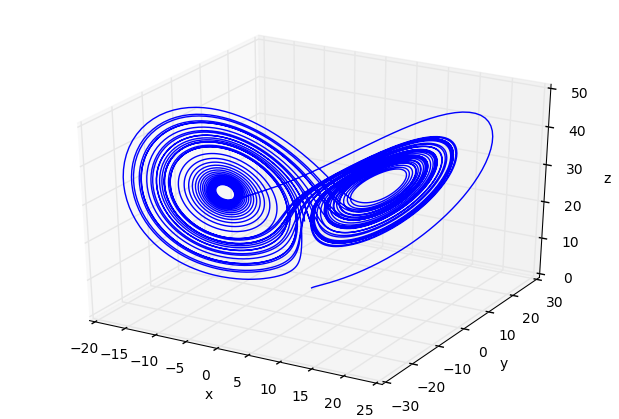fig=plt.figure()
ax=Axes3D(fig)
ax.plot(x2,y2,z2)
ax.set_xlabel('x')
ax.set_ylabel('y')
ax.set_zlabel('z')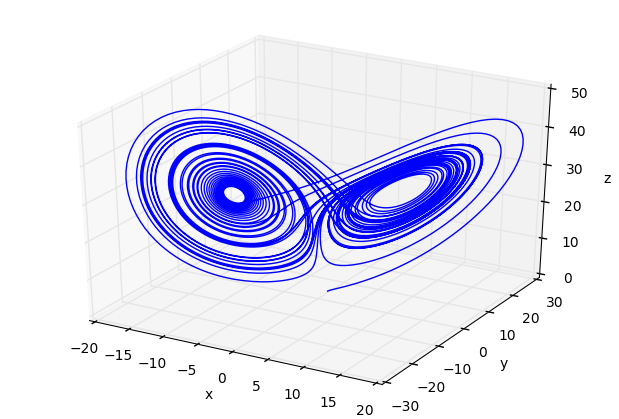You should play around with the time interval (in the definition of varible t) to observe the predictable, followed by chaotic behaviour. ANd play with other parameters.

van der Pol equation

This equation is used as a testbed for numerical software. It is nonlinear, and collapses to a periodic orbit very quickly. We can include information about the Jacobian, the derivative of the vector function $\mathbf{f}$ in the ODE system $\mathbf{y}’ = \mathbf{f}(\mathbf{y},t)$, into the ODE solver, to help it choose appropriate step sizes and reduce errors.

The van der Pol equation is this:

$$y” - \mu(1-y^2)y’ + y = 0$$

with the usual initial conditons for $y(0), y’(0)$. In vector form, we write

$$\mathbf{y} = (y,y’)^T, \qquad \mathbf{f}(\mathbf{y}) = (y’, \mu(1-y^2)y’ - y)^T.$$

The Jacobian is a 2x2 matrix of partial derivatives for $\mathbf{f}$, which is

$$\mathbf{J}(\mathbf{y}) = \left( \begin{array}{cc} 0 & 1 \ -2\mu y * y’ -1 & \mu(1-y^2) \end{array} \right)$$

We set up the code as follows:

def rhsVDP(y,t,mu):
return [ y, mu*(1-y**2)*y - y]

def jac(y,t,mu):
return [ [0,1], [-2*mu*y*y-1, mu*(1-y**2)]]

mu=3 # adjust from 0 to 10, say
t=np.linspace(0,30,10001)
y0=np.array([2.0,0.0])
y,info=odeint(rhsVDP,y0,t,args=(mu,),Dfun=jac,full_output=True)

print("mu = %g, number of Jacobian calls is %d" % (mu, info['nje'][-1]))

plt.plot(t,y)


mu = 3, number of Jacobian calls is 0Try playing with the mu parameter. mu=0 gives the harmonic oscillator. mu=10 starts giving pointy spikes. For mu big, you might want to increase the range of to values, from [0,30] to a larger interval like [0,100]. Etc.

mu=10 # adjust from 0 to 10, say
t=np.linspace(0,30,10001)
y0=np.array([2.0,0.0])
y,info=odeint(rhsVDP,y0,t,args=(mu,),Dfun=jac,full_output=True)

print("mu = %g, number of Jacobian calls is %d" % (mu, info['nje'][-1]))

plt.plot(t,y)

mu = 10, number of Jacobian calls is 0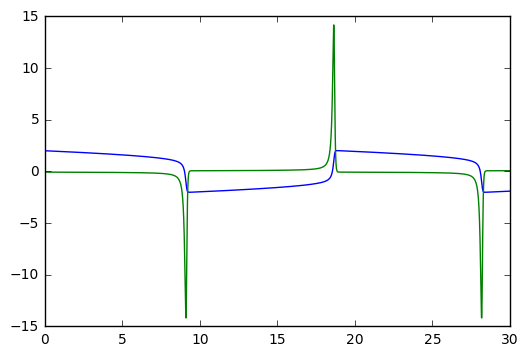Audio Generation

With a few lines of code, we can produce sound and play it.

We will import a few tools to make this possible, including pylab for plotting and numerical work.

%matplotlib inline
from pylab import *
from IPython.display import Audio


We now set up five seconds of sound, sampled at 8000 times per second. We generate two pure tones together at 440 and 442 Hertz. This corresponds to a musical note at A above middle C. The slight difference in frequencies will cause a beating, or fluctuation of the sound at 2 beats per second.

Fs = 8000.0
Len = 5
t = linspace(0,Len,Fs*Len)
f1 = 442.0
f2 = 440.0
signal = sin(2*pi*f1*t) + sin(2*pi*f2*t)

Audio(data=signal, rate=Fs)


We can analyse this signal with the Fourier transform. Plotting, we see the energy is concentrated need 440 Hz (and there is a mirror image in the frequency near 8000-440 Hz).

freqs = t*Fs/Len   # a list of frequencies, in Hertz
fsig = abs(fft(signal))  # Fourier transform of the signal
plot(freqs,fsig)  # amplitude versus frequency

[<matplotlib.lines.Line2D at 0x7fdb59e82358>]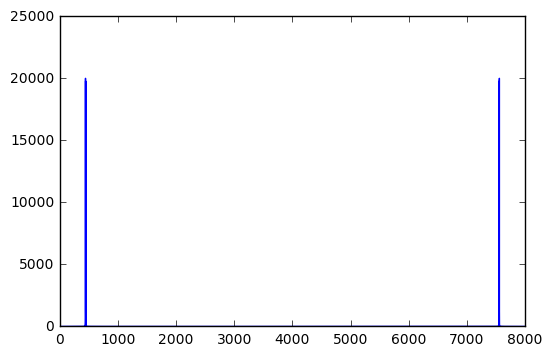By zooming in on the relevant part of the signal, we can see the presence of energy at the two frequencies of 440 Hz and 442 Hz.

plot(freqs[2100:2300],fsig[2100:2300])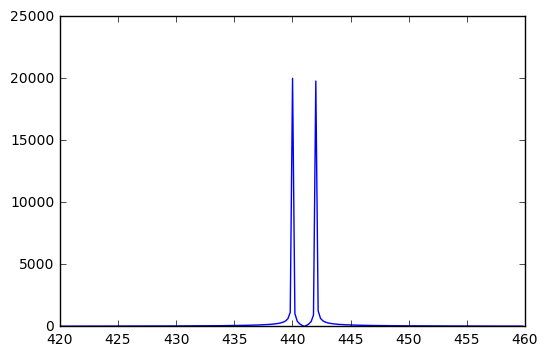Some note on audio in the computer.

The sound we hear with our ears are the rapid vibrations of air pressure on our eardrums, usually generated from vibrations of some object like a guitar string, drum head, or the vocal cords of a human. These sounds are represented as a function of time. In the computer, we represent this function as a list of numbers, or samples, that indicate the value of the function at a range of time values.

Usually, we sample at uniform time intervals. In the example above, we have 8000 samples per second, for a length of 5 seconds. The Shannon sampling theorem tells us that we need to sample at least as fast as twice the highest frequency that we want to reproduce.

Humans with exceptional hearing can hear frequencies up to 20,000 Hz (20 kHz). This suggests we should sample at least at 40,000 samples per second for high quality audio. In fact, a compact disk is sampled at 44100 samples per second, and digital audio tapes at 48000 samples per second.

But, since computer speakers are often of lower quality, we typically sample at lower rates like 8000, 10000, or 22050 samples per second. That give sound that is “good enough” and saves on computer memory.

With just a couple of lines, you can insert a YouTube video into your Jupyter notebook.

from IPython.display import YouTubeVideo
YouTubeVideo('kthi--SH2Nk')   # Counting to 100 in Cree



d3 and fancy user interface

We can use a resource called d3 to great very complex user interfaces in a Notebook.

In this example, a cluster of balls is created that moves around as the computer mouse chases the balls, as displayed here. Click the picture to interact with the example.This code was poached from here: https://github.com/skariel/IPython_d3_js_demo/blob/master/d3_js_demo.ipynb

There are three cells in this example. - the first cell creates a file, which has some html code and javascript to control the balls - the second cell defines Python functions that edie the file, to adjust the number of balls, etc. - the third cell calls the Python funciton f1 that launches the html and javascript.

Here is the first cell.

%%writefile f1.template
<!DOCTYPE html>
<html>
<meta http-equiv="Content-Type" content="text/html;charset=utf-8"/>
<script type="text/javascript" src="https://mbostock.github.io/d3/talk/20111018/d3/d3.js"></script>
<script type="text/javascript" src="https://mbostock.github.io/d3/talk/20111018/d3/d3.geom.js"></script>
<script type="text/javascript" src="https://mbostock.github.io/d3/talk/20111018/d3/d3.layout.js"></script>
<style type="text/css">

circle {
stroke: #000;
stroke-opacity: .5;
}

</style>
<body>
<div id="body">
<script type="text/javascript">

var w = {width},
h = {height};

color = d3.scale.category10();

var force = d3.layout.force()
.gravity(0.1)
.charge(function(d, i) { return i ? 0 : -2000; })
.nodes(nodes)
.size([w, h]);

var root = nodes;
root.fixed = true;

force.start();

var svg = d3.select("#body").append("svg:svg")
.attr("width", w)
.attr("height", h);

svg.selectAll("circle")
.data(nodes.slice(1))
.enter().append("svg:circle")
.attr("r", function(d) { return d.radius - 2; })
.style("fill", function(d, i) { return color(i % {color_count}); });

force.on("tick", function(e) {
i = 0,
n = nodes.length;

while (++i < n) {
q.visit(collide(nodes[i]));
}

svg.selectAll("circle")
.attr("cx", function(d) { return d.x; })
.attr("cy", function(d) { return d.y; });
});

svg.on("mousemove", function() {
var p1 = d3.svg.mouse(this);
root.px = p1;
root.py = p1;
force.resume();
});

function collide(node) {
var r = node.radius + 16,
nx1 = node.x - r,
nx2 = node.x + r,
ny1 = node.y - r,
ny2 = node.y + r;
return function(quad, x1, y1, x2, y2) {
var x = node.x - quad.point.x,
l = Math.sqrt(x * x + y * y),
if (l < r) {
l = (l - r) / l * .5;
node.x -= x *= l;
node.y -= y *= l;
}
}
return x1 > nx2
|| x2 < nx1
|| y1 > ny2
|| y2 < ny1;
};
}

</script>
</body>
</html>


Here is the second cell.

from IPython.display import IFrame
import re

def replace_all(txt,d):
rep = dict((re.escape('{'+k+'}'), str(v)) for k, v in d.items())
pattern = re.compile("|".join(rep.keys()))
return pattern.sub(lambda m: rep[re.escape(m.group(0))], txt)

count=0
def serve_html(s,w,h):
import os
global count
count+=1
fn= '__tmp'+str(os.getpid())+'_'+str(count)+'.html'
with open(fn,'w') as f:
f.write(s)
return IFrame('files/'+fn,w,h)

d={
'width'      :w,
'height'     :h,
'ball_count' :ball_count,

f1(ball_count=50, color_count=17, rad_fac=10, rad_min=3, w=600)